# Multiplying And Dividing Surds Worksheet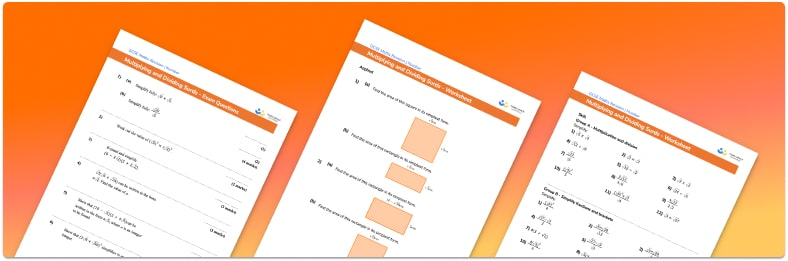• This field is for validation purposes and should be left unchanged.

You can unsubscribe at any time (each email we send will contain an easy way to unsubscribe). To find out more about how we use your data, see our privacy policy.

• Section 1 of the multiplying and dividing surds worksheet contains 36 skills-based multiplying and dividing surds questions, in 3 groups to support differentiation
• Section 2 contains 3 applied multiplying and dividing surds questions with a mix of worded problems and deeper problem solving questions
• Section 3 contains 6 foundation and higher level GCSE exam style multiplying and dividing surds questions
• Answers and a mark scheme for all multiplying and dividing surds questions are provided
• Questions follow variation theory with plenty of opportunities for students to work independently at their own level
• All exam questions created by fully qualified expert secondary maths teachers
• Suitable for GCSE maths revision for AQA, OCR and Edexcel exam boards

### Multiplying and dividing surds at a glance

Surds are numbers left in square root form. Surds are used when detailed accuracy is required for a calculation. Surds are irrational numbers, meaning, when written as decimals, they go on forever without any pattern. Rational numbers can be written as fractions with integer numerators and denominators whereas irrational numbers cannot.

We can multiply and divide surds. When multiplying surds, we use the rule “root a multiplied by root b =  the root of a multiplied by b”. Similarly, when dividing surds we use the rule “root a divided by root b = the root of a divided by b”.

We can also expand single brackets or double brackets that involve surds. This is done by multiplying every term outside of the brackets by every term inside of the brackets and then simplifying the answer by collecting like terms. We can sometimes simplify surds by taking out factors which are square numbers. A surd is in its simplest form when the number under the root sign has no factors which are square numbers.

Looking forward, students can then progress to additional number worksheets, for example aor aFor more teaching and learning support on Number our GCSE maths lessons provide step by step support for all GCSE maths concepts.

## Related worksheets

Rational And Irrational Numbers Worksheet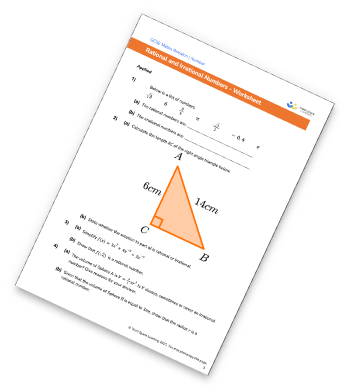Surds Worksheet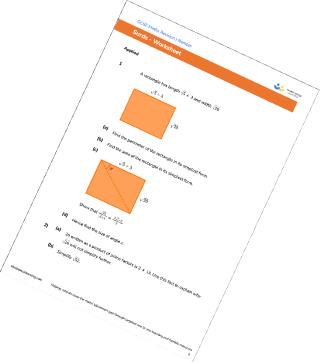Rationalise The Denominator Worksheet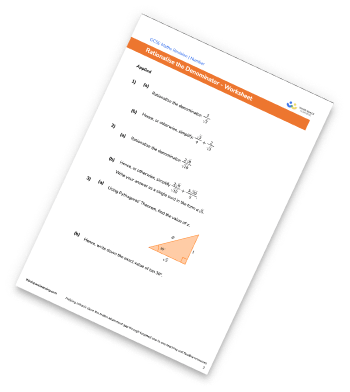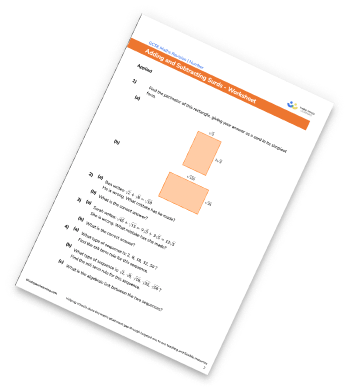## Do you have KS4 students who need more focused attention to succeed at GCSE?There will be students in your class who require individual attention to help them succeed in their maths GCSEs. In a class of 30, it’s not always easy to provide.

Help your students feel confident with exam-style questions and the strategies they’ll need to answer them correctly with our dedicated GCSE maths revision programme.

Lessons are selected to provide support where each student needs it most, and specially-trained GCSE maths tutors adapt the pitch and pace of each lesson. This ensures a personalised revision programme that raises grades and boosts confidence.

Find out more